# Part Seven: Mythic Theory of Everything

`Having an explanation,Is not the same thing as having a solution.`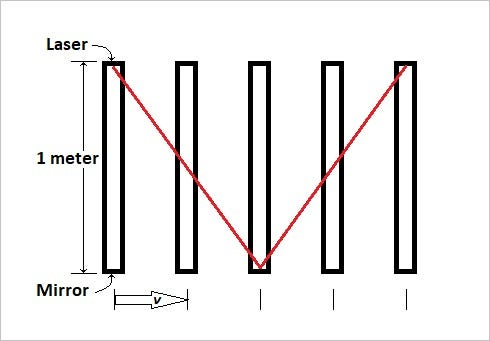Illustration of a One Meter Tall Laser Clock in Motion

## Foreword

The Mythic Theory of Everything (MToE) is a fabricated theory created as a parody of modern physics. It has been refined to be irritating to all schools of study, but if I have failed to include anyone, please advise and I will make amends. For some it might be seen as pseudo-science, although at this time there is no advocate presenting it as actually being true. I freely agree that it is nonsense and it should not be taken seriously. In that capacity, it can never be anything more than just junk science. Ironically, it demands explicit adherence to the rules that it is built on, so it makes a great thought experiment. If nothing else, it can provide a completely different (non-medicated) perspective of some aspects of physics and something like that can sometimes actually be inspirational. This essay addresses how Special Relativity looks when viewed with the MToE.

This article is part of the series called the Mythic Theory of Everything. If you view my Lists and About data, all the other articles can be seen.

## Chapter 1 — Background

Do not under any circumstance study the MToE as actually theory. Keep in mind that the proper use of the MToE is to educate from a devil’s advocate position. For example, if I disagree with an aspect of modern physics, e.g. photons, rather than rant and rave about the subject and disavow the volumes of scientific literature, I simply ask the reader to explain why the MToE is wrong. Admittedly, the MToE concept is ridiculous, but it still demands thoughtful rebuttal to dismiss.

The first concept of the MToE states that giant filament loops fill up our universe. The filaments themselves are super strong, immensely long, very thin, and completely friction-less. These loops exist in a jumble that defines our universe. Other jumbles of filaments might well constitute other universes that we can not detect.

The second concept of the MToE states that all energy is transmitted though the filaments that make up the universe. As such, all treatment of electromagnetic phenomena must be viewed as actual waves and vibrations in these filaments.

The third concept states that mass is a stored form of energy when a loop is created in a one of these filaments. The loop can roll along the filament and represents an elemental particle. The loop also rotates around the filament to impart electromagnetic properties.

Details of the original MToE can be found at A Peek Into The Future Of Physics.

See the Wikipedia article Special Relativity (17 June 2022, at 10:23 UTC.)

`... theory is based on two postulates:1.  The laws of physics are invariant (that is, identical) in all inertial frames of reference (that is, frames of reference with no acceleration).2.  The speed of light in vacuum is the same for all observers, regardless of the motion of the light source or observer.Time dilationThis shows that the time (Δt′) between the two ticks as seen in the frame in which the clock is moving (S′), is longer than the time (Δt) between these ticks as measured in the rest frame of the clock (S).Length contractionThis shows that the length (Δx′) of the rod as measured in the frame in which it is moving (S′), is shorter than its length (Δx) in its own rest frame (S).`

Also see the Wikipedia article on Lorentz Transformations (4 July 2022, at 16:16 UTC.)

`… where v is the relative velocity between frames in the x-direction, c is the speed of light, and (lowercase gamma) is the Lorentz factor.       γ = 1/√[1− v^2/c^2]`

The Lorentz Factor is used to calculate Time Dilation and Length Contraction in Special Relativity. A table of some selected factors is provided at the end of the article in Appendix A.

## Chapter 2 — Review of Conventional Special Relativity

Although an understanding of frames of reference is integral to Relativity, I will simplify my discussion by using only two reference frames. The first, our stationary observer, is located on a motionless platform in deep space. The second, our traveler, is riding in a transparent spaceship traveling on a straight path that goes past the platform. Our “observations” will of course be hypothetical as we are ignoring many nuances that might arise in a real world situation, e.g. micro-gravity, the speed of information, observational precision, the influence of weak electromagnetic radiation, and so forth.

For the convenience of illustration, I will be using an imaginary time interval that I call a Tick. One Tick is equal to the time that it takes for light to travel one meter.

`One Tick = 1 m/c = 1 m/(299,792,458 m/s)= 3.3356e-9 seconds.`

Our observer and our traveler will each have identical clocks that are one meter long. See the illustration at the top of this article. Each clock has a laser contained in a vacuum that bounces off a mirror at one end. When the beam returns to the laser end, then a cycle is complete and the clock shows 2 Ticks, one outgoing transit and one incoming transit. The postulates of Special Relativity tell us that these clocks will always record the same local value regardless of the orientation or the velocity of the user, i.e. each person will always see 2 Ticks per cycle of their clock regardless of where they are or how fast they are traveling.

Thus, we begin the examination of Time Dilation. When the spaceship is accelerated up to a constant velocity we notice something interesting. The beam of light is not going straight to the mirror, but is traveling on a slope toward the mirror. The stationary observer on the platform can see this, but the traveler in the ship only sees the beam bouncing up and down. By the time we reach the right side of the illustration, the traveler is still only showing 2 Ticks on the spacecraft clock, where the stationary observer is showing more. As it turns out, we have an equation to quantify this observation.

Time_Dilation = Travel_Time × γ

If the spaceship is traveling approximately 86.6% of the Speed of Light, then γ = 2 and our stationary observer will see the beam stretched out to around 2 meters on each transit and will count 4 Ticks on the platform clock. Consequently, the stationary observer on the platform has experienced a Time Dilation and is aging twice as fast as the traveler on the spacecraft.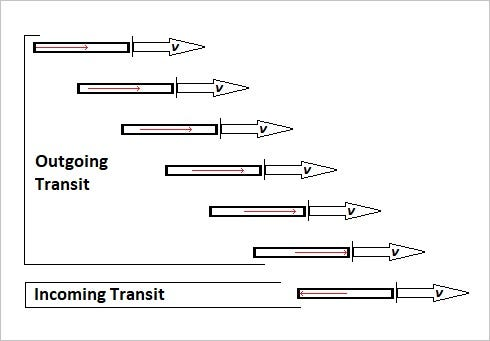Illustration of a Moving One Meter Long Laser Clock

Length Contraction is simply an extension of Time Dilation, but it is a little tricky. If we turn our clock on its side so that it is parallel to the direction of motion, we see that again the spacecraft clock shows 2 Ticks. We quickly note that it takes longer to chase down the mirror than it takes to return to the laser end, but the traveler does not realize this because of a principle called the Relativity of Simultaneity. It is important to Relativity, but it is beyond what I want to present here. In a condensed version, if you are moving, i.e. a traveler, you can not tell the timing of events that are obvious to a stationary observer. Check out the Wikipedia article at Relativity of Simultaneity (26 July 2022, at 17:37 UTC).

Since we can not look at the traveler’s experience, all we can do is ask “Will the stationary observer still see 4 Ticks?” The problem that the length of the outgoing transit is considerably longer than the incoming transit makes it difficult to easily judge graphically what the stationary observer is seeing.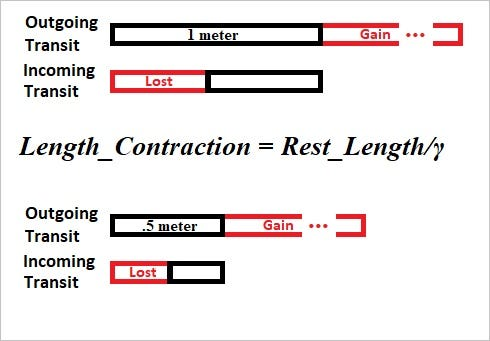Illustration of the Correction for Length

If we look at what is happening, we see that for the outgoing transit the Clock_Length = 1 meter + Extra_Gain and in the return transit the Clock_Length = 1 meter − Extra_Lost. Since we know the velocity of the spacecraft, we can figure out what the extra lengths are and thus know what the stationary clock will register. For those that want to see the math, see Appendix B. In summary, if we keep the clock size at one meter, we require 8 Ticks on the stationary clock; however, if we use the following equation, we shorten the transits and the stationary observer will go back to seeing only 4 Ticks.

Length_Contraction = Rest_Length/γ

In this case, we see that instead of multiplying the distance by the Lorentz Factor, we divide it. So, if our factor is 2, then the inverse is ½. Thus, the stationary observer will see the 1 meter moving clock to be only ½ meter long. The important thing to note in this case is that Length Contraction is dependent on Time Dilation, i.e. we use the 4 Ticks that stationary observer sees instead of the 2 Ticks that the spacecraft clock is recording to derive the Length Contraction.

In summary, Time Dilation affects the stationary observer and Length Contraction affects the traveler. If you should be lucky enough (or unlucky enough) to wait at a train crossing, you can see an illusion that resembles Relativity. The passing cars on the train will appear shorter as the train moves faster and your time at the crossing will seem to slow down and last forever.

## Chapter 3 — Filament Relativity

Ok, it is time to talk about the MToE. On first principles, Special Relativity considers the Speed of Light as invariant and Time and Space as malleable. The MToE considers Time and Space as invariant and the Speed of Light as changeable. How then does this work?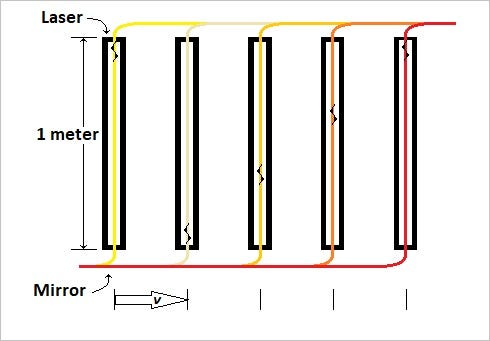Illustration of a Moving One Meter Long Filament Clock

The MToE clock has a group of filaments trapped in between exclusion bubbles, i.e. atomic shells, at both ends of the clock. These filaments run the length of the clock and carry the laser energy wave pulses. At rest the wave pulse travels up and down at the Speed of Light just like we have seen before. The filament is actually stationary with regard to the universe, however, it must quickly move from the top to the bottom of the clock when the spacecraft moves forward. This will create a condition where the wave pulse on the filament is moving faster than the Speed of Light. If this seems unbelievable, think of a guitar moving along a conveyor belt. If there is a vibration pulse on a string, it will technically move faster than what is mechanically possible because it is being carried by the conveyor. When the pulse bounces off the mirror, it will reverse its direction and it will be traveling slower than the Speed of Light as it flows upstream.

If the filament is threaded from bottom to top, it will work in the opposite fashion, aiding the up direction and hindering the down direction. The particular direction that a filament will assume is completely random, so half the time it helps and half the time it does not. If the MToE were actually true, the waves would be traveling simultaneously in both orientations. Regardless, the average speed would of course always be the Speed of Light.

I will skip the horizontal clock illustration, because it is easy to see it moves alternately upstream and downstream along a stationary filament, benefiting from and fighting against the filament and of course having the average Speed of Light.

Consequently, Time Dilation and the related Length Contraction are not features of the MToE. Knowing that high speed particle experiments have confirmed these phenomena for decades, one might think that the MToE is dead on the spot. Sadly, as we will see, that is not the case.

## Chapter 4 — The Mass Loop Card

The mass loop is the basic particle in the MToE universe and this fact is the “Get out of Jail Free Card” for this situation. Our first stop on this section is the concept of Relativistic Mass. See the Wikipedia article at Mass in Special Relativity (25 July 2022, at 01:28 UTC).

`The word mass has two meanings in special relativity: invariant mass (also called rest mass) is an invariant quantity which is the same for all observers in all reference frames, while the relativistic mass is dependent on the velocity of the observer.[That confuses me as well.  I think they mean velocity relative to the observer, because that is how it appears later on.]For a particle of finite rest mass m moving at a speed v relative to the observer, one findsm_rel = m  /√[1− v^2/c^2]`Mass Loop Increases Mass as it Rolls Faster

If we think of the mass loop in the MToE as a vehicle tire, then the mass loop moves by rolling along the filament. It takes energy to move the loop faster. As it increases speed the trans-mass of the filament that makes the loop is flung outward and the loop expands much like an unconstrained vehicle tire would. This increases the size of the mass loop and consequently the mass (and energy) contained in the loop. (Much as I disdain the MToE, I do like this representation of E = mc².)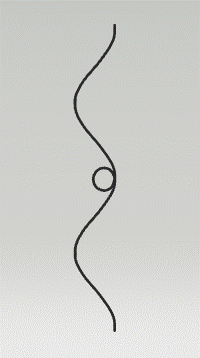Mass Loop Rotates while Moving along a Wave

From the above illustration, we note that the mass loop is not only rolling along the filament, but it is also rotating around the filament. As it increases in mass, if nothing increases the angular momentum, then the speed of this rotation is slowed down. Since virtually all clock and metabolic processes depend on the angular momentum of atoms and particles, guess what, we now have the MToE version of Time Dilation. Time itself remains invariant, but clock time is slowed down.

One might assume that with clock time shown to be slowed down, it might seem reasonable that the original arguments for Length Contraction should still be valid for the MToE. We can not do that because if we make Time invariant, then the spacial dimensions logically should also be invariant; otherwise, we violate our own premise.

Continuing, we note that the effective zone of a particle’s influence is governed by the de Broglie wavelength function. In simple terms, as a particle moves faster, the de Broglie wavelength shrinks much as we would expect if it were affected by Length Contraction. Now, to be fair, this is not an accepted concept in the physics world, but the MToE is not an accepted concept either, so here is the blurb.

If we exam the de Broglie wavelength equation we find an odd function: Wavelength = Planck_Constant / (m × v). When a particle is at rest v = 0, the function trends toward infinity at one end, but at the fast end, we can actually set v = c and get a finite result. Since we know that can not be correct, we must use the relativistic de Broglie wavelength: Wavelength = Planck_Constant / (γ × m × v) where γ is the Lorentz Factor = 1/√[1− v²/c²]. We now see the high velocity side trend toward zero and the whole plot becomes a stretched out and side wise “S.”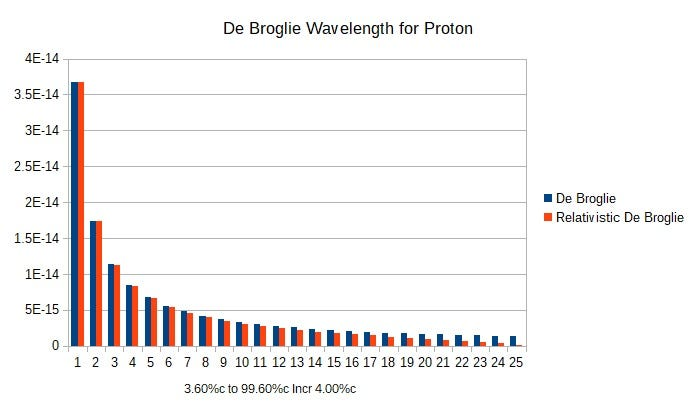Full Range Graph of De Broglie Wavelength for a Proton

We obviously can not compare the full range of de Broglie wavelengths to the Length Contraction function. So, we will assign the baseline length for Length Contraction to a specific de Broglie wavelength and watch the Length Contraction plot against the relativistic de Broglie curve originating at that point. I will arbitrarily pick 75%c.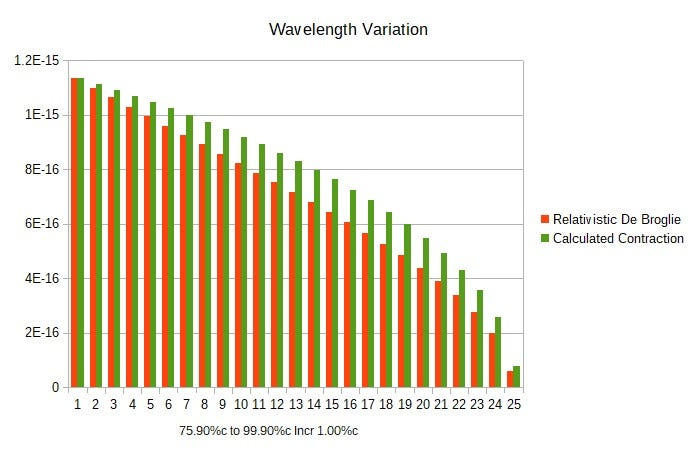Limited Range Graph of De Broglie Wavelength compared to Length Contraction

In all cases the relativistic de Broglie wavelength is actually equal to or smaller than the calculated Length Contraction. Actually this works for any velocity selected. To be clear, this does not make the de Broglie wavelength equivalent to Length Contraction, but it does not invalidate the concept. Thus, if we accept the concept as true, all experiments would indeed demonstrate at least the minimum expected Length Contraction.

## Chapter 5 — Crazy Experiment Time

If the Relativity of Simultaneity does not make it impossible, I have a simple test for Special Relativity and the MToE, but I am not sure whether we have the technology or the even the measurement accuracy to do it, or for that matter whether someone has done it already. I think that if it can be done, it should pretty much put the DOA and RIP abbreviations into close association with the MToE.

For this test, we hook two synchronized high accuracy clocks together separated by a precisely determined distance. Environmentally isolate the systems and periodically during the day simultaneously beam a laser from each toward the other and measure the individual one way transit times. Over the course of a day, the laboratory will be reoriented due to the earth’s rotation, so that two axes can be measured at 90 degrees to each other at roughly six hour intervals with out any need to move the equipment itself. To measure the last axis, the laboratory equipment can be rotated 90 degrees, i.e. the initial orientation of East-West can be changed to North-South. Even if it is not possible to execute this test, it still should make a great paper just explaining why it can not be done.

## Chapter 6 — Conclusions

The inclusion of the de Broglie wavelength as an explanation for Length Contraction is likely to cause some serious students of physics to have a conniption. In truth, I would have no problem if someone can propose a more suitable explanation, but without a plausible phenomena, we must fall back to the age old “warping of space-time fabric.” This of course begs the question, when two Frames of Reference collide, which formulation of time and length do we use to calculate the result? Beware the answer of both, each effecting the initial contents of their respective frames, because you would be suggesting that time and length are invariant after all. Although de Broglie can be argued one way or another, it does pose an interesting question of whether the de Broglie wavelength should be accounted for in some fashion by Special Relativity?

Although the MToE might have a different perspective, it certainly does not invalidate the theory of Special Relativity. It does, however, offer an outrageous concept, since Time and Space become invariant, the Speed of Light becomes variant and mortal. My dilemma becomes “Should I believe in conventional physics and never have any hope of a hyper-drive or gamble on the MToE and have the possibility of a hyper-drive?”

Tempting as it may be, the truth will eventually prevail and I think the odds are against the MToE winning. Of course, I tend to be famously bad at picking horses, so I might be wrong and totally delighted with the consequences of my failure!

## Appendix B — Math for Length Contraction

`Using Gt and Lt as the extra times, we note that Gt + Lt = Number of Ticks.For Extra_Gain: c × Gt = 1m + v × Gtand for Extra_Lost: c × Lt = 1m − v × Lt.Solving gives two equations:  Gt = 1m/(c − v) and Lt = 1m/(c + v).Combining gives us Number of Ticks = 1m/(c − v) + 1m/(c + v).Test without Length Contraction.Do 4 Ticks = 1m/(c − v) + 1m/(c + v)?4 Ticks = 1m/(c − 0.866c) + 1m/(c + 0.866c)4 Ticks = 1m/.134c + 1m/1.866c4 Ticks = 7.4626 m/c + 0.5359 m/cSince 1 Tick = 1 m/c, we can substitute4 Ticks = 7.4626 Ticks + 0.5359 Ticks4 Ticks ≠ 7.9985 Ticks`

Oops, this does not work, but note that the factor is around twice as large as expected. Knowing that this equation can not be resolved and knowing how to get the correct answer, we use the transform:

Length_Contraction = Rest_Length/γ

`Since γ = 2, we have Length_Contraction = 1m/2 = ½ meter:Do 4 Ticks = 0.5m/(c − v) + 0.5m/(c + v)?4 Ticks = 0.5m/(c − 0.866c) + 0.5m/(c +.866c)4 Ticks = 0.5m/.134c + 0.5m/1.866c4 Ticks = 3.7313 m/c + 0.2679 m/cSince 1 Tick = 1 m/c, we can substitute4 Ticks = 3.7313 Ticks + 0.2679 Ticks4 Ticks = 3.9992 Ticks`

Ignoring rounding errors, it works. For those interested, here is the link to the actual math formula derivation.

--

--